IF YOU CAN SEE THIS go to /sfa/Nuprl/Shared/Xindentation_hack_doc.html

### Y-combinator Continued - from Recursion via the Y-combinator

Now, let's see how to exploit Y(f) *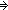f(Y(f)) to express recursive function definitions, which are not primitive in Nuprl. A general form of a one-place recursive function definition would be

f(z) == G(zx.f(x))

which indicates that in evaluating f(z), not only does the function G(zx.f(x)) have access to the argument z that was supplied in f(z), but also it may further apply function f(x) to any new arguments at all; thus, recursive calls to f(x) may be made, for various values of x, as part of evaluating f(z).

However, in Nuprl, such recursive definitions are not built-in, they are not primitives. The primitive Operator Definitions of Nuprl must not use the defined operator in its own expansion. So, how do we implement "f(z) == G(zx.f(x))"?

f(z) == Y((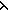h,z. G(zx.(h(x)))),z)

Notice that in this definition, the operator f(?) does not occur on the right hand side, so the definition is an ordinary one, rather than a recursive one. The recursive "behavior" is a result of using the Y-combinator, as illustrated below. Note that we're not just looking at Y applied to one argument "h,z. G(zx.(h(x)))" but its further application to another argument u in order to see how f(u) would be evaluated (recall that "g(x,y)" means "(g(x))(y)"; see Multi-Argument Functions).

Y((h,z. G(zx.(h(x)))),u)
*(h,z. G(zx.(h(x))))(Y(h,z. G(zx.(h(x)))),u)
*G(ux.(Y((h,z. G(zx.(h(x)))),x)))

Further evaluation depends upon how G(?x.?) is evaluated. Observe that "Y(h,z. G(zx.(h(x))))" has been replicated down inside of the instance of G(?x.?), and that how it gets further applied is up to G(?x.?).

Returning to our definition of factorial, introduced in Recursive Functions,

Def x! == if x=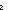0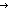1 else x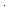(x-1)! fi  (recursive)

is actually an improved representation of the actual content of the definition, which is:

x! == Y((sfa_doc_factorial,x. if x=01 else xsfa_doc_factorial(x-1) fi),x)

The recursive form was entered by the user, and this primitive form was automatically generated and stored as the "official" definition. The variable name "sfa_doc_factorial" was selected automatically by the system, using the name assigned to this factorial operator by its creator (sfa).

Note: Although we type applications of Y to functions, as we did for Thm*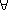x: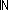x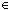, we don't assign Y itself a function type. For a discussion of how this could be accomplished see Typing Y.

(Feb 2001 - sfa )